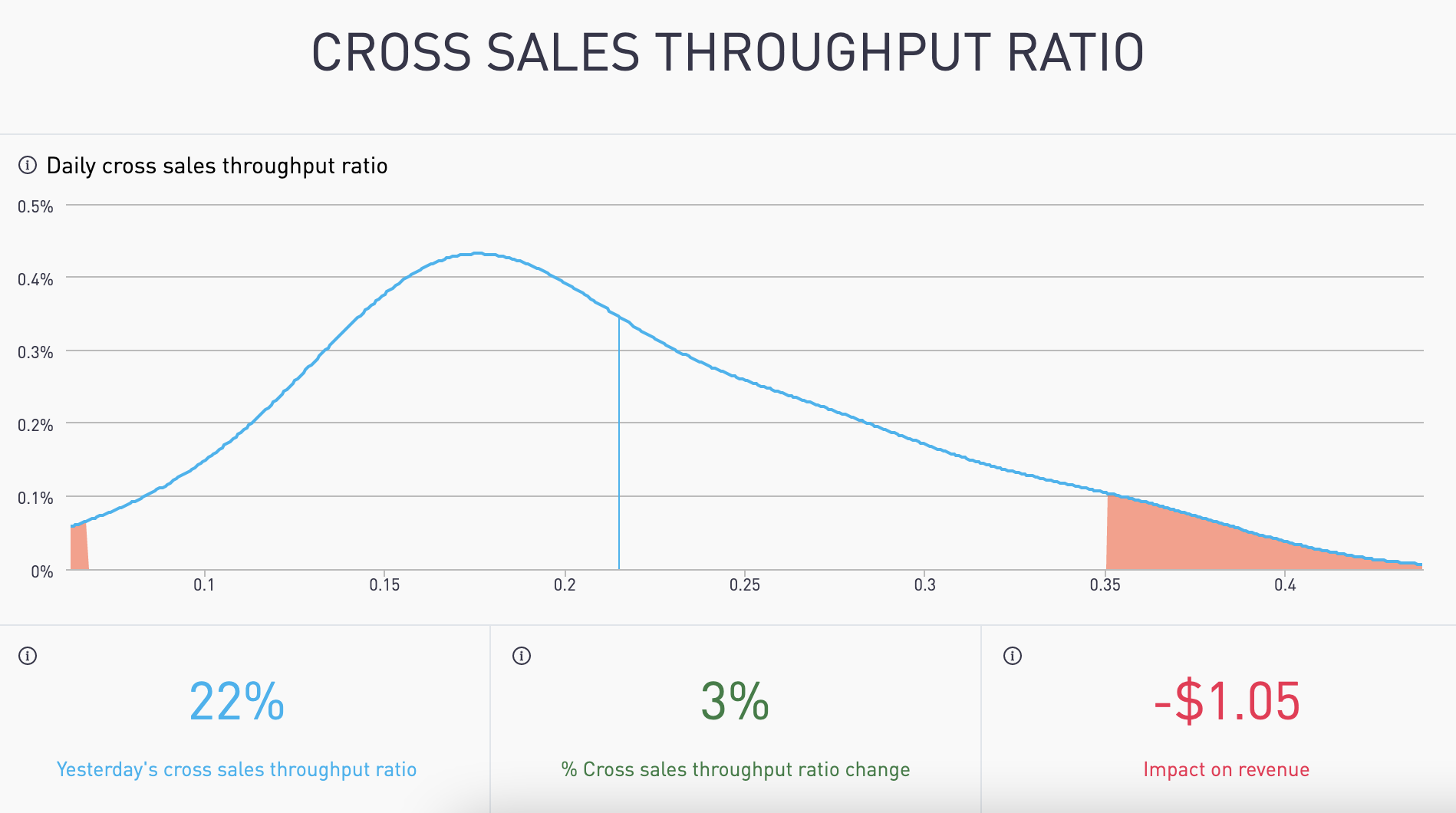# Cross Sales Throughput Ratio

See yesterday's cross-sales throughput ratio. What impact did it have on your revenue?## Is it today's result common? Is it rare?

Density charts help you to see beyond simple averages to know if you need to take action. Decision-makers within companies have to focus on what matters. A simple average can't tell you when something is unusually good or bad. The density chart tells you how often something happens.

(density charts to detect trends) A density chart is the distribution of data over a continuous interval or time period.

# The Terms

Yesterday's Cross Sales Ratio

As a seller, the numbers of cross-sales subscriptions generated from your sales divided by your total sales for yesterday.

% Cross Sales Ratio Change

The change in yesterday's cross-sales ratio from the average of the last 4 weeks same day as yesterday cross-sales ratio as a percentage.

Impact on Revenue

The impact change in the cross-sales ratio has on total revenue.

# The Math

Yesterday's Cross Sales Ratio

Total cross-sales divided by total signups for yesterday.

% Cross Sales Ratio Change

If yesterday was "Sunday", then the yesterday cross-sales ratio is compared with the average of previous 4 "Sunday's cross-sales ratio and represented as a percentage.

Impact on Revenue

Calculated by finding the regression coefficient for cross-sales ratio using linear regression multiplied with the change in the cross-sales ratio(Eg-The difference between Sunday's cross-sales ratio & the previous 4 Sunday's cross-sales ratio multiplied with the regression coefficient), represented as a US dollar amount.

# The Visuals

X axis (or horizontal axis)

Cross-sales ratio

Y axis (or vertical axis)

How often a particular cross-sales ratio occurred during the period. This is also known as frequency.

Blue Curved Line

The frequency of each cross-sales ratio during the period shown on a smoothed line.

Blue Vertical Line

Yesterday's cross-sales ratio.